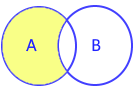# difference() method in Python set

All set methods

Returns a new set with difference elements of main set only ( after removing common elements) . Original sets remains unchanged.

## difference using - operatorAll difference elements of first set
Using minus ( - ) operator
``````A={1,2,3}
B={3,4,5}
print(A - B)``````
Output
``{1,2}``

## Using type()

We can check the output by using type()
``````A={1,2,3}
B={3,4,5}
x=A - B
print(type(x))``````
Output
``<class 'set'>``

## Using difference() method

``````A={'a','b','c'}
B={'a','y','z'}
print(A.difference(B))``````
Output
``{'c', 'b'}``

## Using more than one sets

We can use any number of sets with difference()
``````A={'a','b','c'}
B={'a','y','z'}
C={'a','b','l'}
print(A - B - C)``````
Output
``{'c'}``

## difference() method

Using string ( iterable object ) with difference() method
``````A={'a','b','c','x','y'}
B='Alex'
print(A.difference(B))``````
Output
``{'a', 'c', 'y', 'b'}``
Using list with difference() method.
``````A={'a','b','c'}
B=['a','x','y']
print(A.difference(B))``````
Output
``{'c', 'b'}``
Below code will generate error.
``print(A - B)``
`` TypeError: unsupported operand type(s) for -: 'set' and 'list'``

## difference_update()

In all above code by using difference() we created a new set. By using difference_update() method we can change the original set with difference elements.

Subscribe to our YouTube Channel here

## Subscribe

* indicates required
Subscribe to plus2netplus2net.com